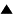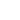Adaptive syser: a model of a hypothetical prebiological control system
We describe here the model of an adaptive syser , which can modify its behavior in accordance with external environmental change. We consider also the mini-syser and compare selective abilities of both sysers.

The mini-syser (Fig. 1a) is a very simple syser, it includes only such macromolecules, which are necessary and sufficient for self-reproduction, namely, the matrix I , the replication enzyme E1 , and the translation enzyme E2 .

The adaptive syser (Fig. 1b) includes two additional enzymes: the regulatory enzyme E3 and the adapting enzyme (adapter) E4 . The regulatory enzyme E3 recognizes the environment state and "turns on" or "turns off" the synthesis of the adapter in accordance with the environment changes.Fig 1. The schemes of mini-syser (a) and adaptive syser (b). I is the polynucleotide matrix, E1 , E2 , E3 , and E4 are replication, translation, regulatory, and adapting enzymes, respectively.

We suppose that there are two types of external environment, A and B. The environment A is a usual one, in which both sysers are able to reproduce themselves. The environment B is unusual, in which the macromolecular synthesis takes place only in the adaptive syser. The regulatory enzyme E3 in the adaptive syser is synthesized with a small rate in both A and B environments, it recognizes the environment state and "turns on" the synthesis of the adapter E4 in the environment B and "turns off" this synthesis in the environment A. The adapter E4 provides the macromolecular synthesis in the adaptive syser in the environment B.

For example, we may imply that the environment A corresponds to the usual syser's "food", usual powerful chemical substrate SA , and the environment B corresponds to the usually "uneatable" chemical substrate SB , which can be transformed into "eatable food" SA by means of the adapter E4. "For the sake of economy", it is natural to synthesize the adapter only then, when it is really needed, i.e. only in the environment B. To recognize the environment state, the regulatory enzyme E3, which is synthesized always, but "economically" (with a small rate), is included in the syser structure. The scheme of this molecular control is similar to the classical model by F. Jacob and J. Monod .

To describe quantitatively the syser's features, we use the following assumptions: 1) the different sysers are placed into separate coacervates; 2) any coacervate volume is proportional to the number of macromolecules inside it. From these assumptions we obtain the following equations:
 dNi /dt = Vfi , V= c-1Si Ni , xi = Ni /V , (1)

where Ni and xi are the number of molecules and the concentration of i-th macromolecules in a given coacervate, respectively; V is coacervate volume; fi is the synthesis rate i-th macromolecules; c is the constant total concentration of macromolecules in a coacervate (c = Si xi = const); here the index i = 0 refers to matrix I, and other i (= 1,2,3,4) refer i-th enzymes (E1 , E2 , E3 , E4), respectively. We define the synthesis rates as follows.

For the mini-syser we set:
 f0 = a0 x0 x1 , fi = ai x0 x2 , i = 1,2, in environment A , (2a)
 f0 = f1= f2 = 0 , ; ; in environment B . (2b)

For the adaptive syser we set:
 f0 = a0 x0 x1 , fi = ai x0 x2 , i = 1,2,3, in environment A , (3a)
 f0 = b0 x0 x1 , fi = bi x0 x2 , i = 1,2,3,4, in environment B . (3b)

Here ai and bi are synthesis rate parameters. Eqs. (2), (3) state that the matrix/enzyme synthesis rate is proportional to the matrix and replication/translation enzyme concentrations.

From (1) we obtain:
 dxi /dt = fi - xi c-1 Sj fj , (4)
 dV/dt = V c-1 Sj fj . (5)

According to (2) - (4), the concentration dynamics in a particular syser is described by nonlinear ordinary differential equations, which were analyzed  by usual qualitative methods. The analysis showed that the macromolecules concentrations xi converge to the equilibrium stable state x0 = {x0i }. In the environment A the values x0i are expressed as (for both sysers):
 x00 = c a0 a1 D , x0i = c ai a2 D , D = [a0a1 + a2 (a1+ ... + an)]-1, i = 1, ..., n, (6)

where n is a number of enzymes in a considered syser (here we set a4 = 0) . For the adaptive syser in the environment B, the equilibrium concentrations x0i are also determined by Eq. (6) after substitution bi instead ai .

According to equation (5), the coacervate volume rates are proportional to selective values:
 W = c-1 Sj fj . (7)

Analogously to hypercycles, we can consider the competition of mini-syser and adaptive syser explicitly, supposing that the total coacervates volume for both types of sysers should be constant. During competition, a syser, having the maximal selective value W , is selected. Assuming small convergence time to the equilibrium state x0 and substituting values x0i , determined by formulas (6), into (7) , we obtain the selective values of the considered sysers. For the mini-syser we have
 WMini_A = c a0 a1 a2 [a0a1 + a2 (a1+ a2 )]-1 , WMini_B = 0, (8)

in the environments A and B, respectively. For the adaptive syser the corresponding selective values are expressed as follows:
 WAdaptive_A = c a0 a1 a2 [a0 a1+ a2 (a1+ a2 + a3)]-1 , WAdaptive_B = c b0 b1 b2 [b0 b1 + b2 (b1+ b2 + b3 + b4)]-1 . (9)

These expressions show, that in the environment A , the mini-syser has a selective advantage with respect to the adaptive one, because WMini_A is always greater, than WAdaptive_A . Such a disadvantage of the adaptive syser is due to the necessity to synthesize always the additional regulatory enzyme E3 . The disadvantage is small, if regulatory enzyme synthesis rate is small (a3 << a1, a2). Obviously, the adaptive syser is preferable in the environment B.

If the environment states (A and B) are intermittent, we can introduce the effective selective values of the considered sysers:
 WMini = (1 - PB) WMini_A , (10)

where PB is the probability of the environment B . The adaptive syser has a selective advantage with respect to the mini-syser, if WAdaptive > WMini . From expressions (8)-(11) we can see that the adaptive syser is significantly preferable, if the regulatory enzyme synthesis rate is small (a3 << a1, a2) and the macromolecular synthesis rate in the environment B as well as the probability of this environment are sufficiently large (bi ~ ai and PB ~ 1).

Thus, the adaptive syser does have a selective advantage with respect to the mini-syser, however not always, but only if the "expenses", needed to support the molecular control system operation, are sufficiently small.

Conclusion. The control system of the adaptive syser could be the first control system, which was "invented" by biological evolution. The adaptive syser model demonstrates quantitatively, that the new evolutionary invention has selective advantages, if "invention profits" are greater than "invention expenses".

References:

1. V.G.Red'ko. Biofizika. 1990. Vol. 35. N.6. P. 1007 (In Russian).

2. F.Jacob and J.Monod. J. Mol. Biol. 1961. Vol. 3. P. 318.

 HomeMetasystem Transition TheoryEvolutionary TheoryMathematical Modeling of EvolutionModels of molecular-genetic systems originSysers Up Prev.Next Down